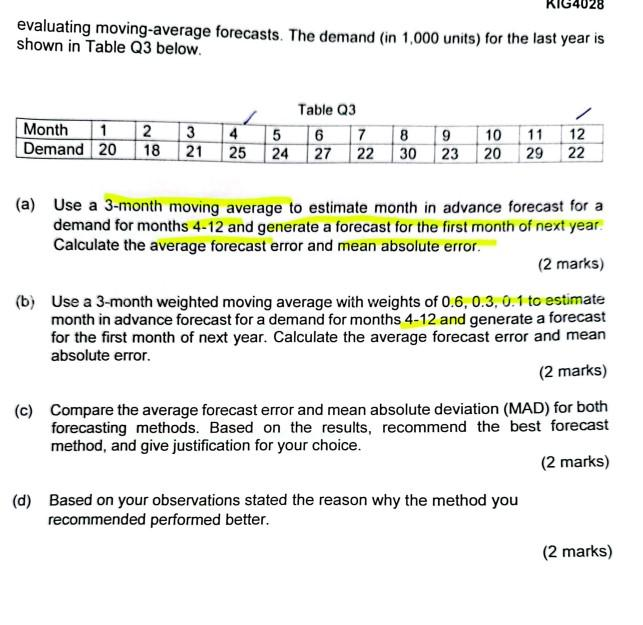Home / Expert Answers / Operations Management / evaluating-moving-average-forecasts-the-demand-in-1-000-units-for-the-last-pa955

# (Solved): evaluating moving-average forecasts. The demand (in 1,000 units) for the last ...​​​​​​​

evaluating moving-average forecasts. The demand (in 1,000 units) for the last year is shown in Table Q3 below. Table O3 (a) Use a 3-month moving average to estimate month in advance forecast for a demand for months 4-12 and generate a forecast for the first month of next year. Calculate the average forecast error and mean absolute error. (2 marks) (b) Use a 3-month weighted moving average with weights of to estimate month in advance forecast for a demand for months and generate a forecast for the first month of next year. Calculate the average forecast error and mean absolute error. (2 marks) (c) Compare the average forecast error and mean absolute deviation (MAD) for both forecasting methods. Based on the results, recommend the best forecast method, and give justification for your choice. (2 marks) (d) Based on your observations stated the reason why the method you recommended performed better. (2 marks)

We have an Answer from Expert

A)

To calculate the 3-month moving average forecast for months 4-12 and the forecast for the first month of the next year, follow these steps:
Compute the 3-month moving average for each month by averaging the demand for the current month and the two preceding months. Start from month 3 since we need data for the first forecast in month 4.

2. Calculate the forecast for the first month of the next year by taking the moving average of the demand for months 10, 11, and 12.

Forecast for the first month of next year = (20 + 29 + 22) / 3 = 23.67

3. Calculate the forecast errors by subtracting the actual demand from the corresponding forecasted values for months 4-12.

4. Calculate the average forecast error by summing up the forecast errors and dividing by the number of forecasts.

Average Forecast Error = (5.33 + 2.67 + 3.67 - 3.33 + 5.67 - 3.33 - 5 + 4.67 - 2) / 9 = 7.67 / 9 = 0.8522 (rounded to 4 decimal places)

5. Calculate the mean absolute error by taking the absolute value of each forecast error, summing them up, and dividing by the number of forecasts.

Mean Absolute Error = (|5.33| + |2.67| + |3.67| + |-3.33| + |5.67| + |-3.33| + |-5| + |4.67| + |-2|) / 9
= (5.33 + 2.67 + 3.67 + 3.33 + 5.67 + 3.33 + 5 + 4.67 + 2) / 9 = 36 / 9 = 4

Therefore, the average forecast error is 0.8522 (rounded to 4 decimal places), and the mean absolute error is 4.

We have an Answer from Expert